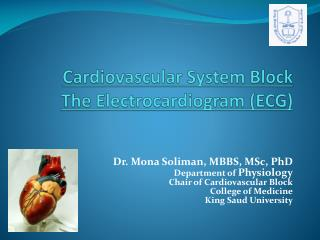DownloadDownload PresentationCardiovascular System Block The Electrocardiogram (ECG)

# Cardiovascular System Block The Electrocardiogram (ECG)

Télécharger la présentation## Cardiovascular System Block The Electrocardiogram (ECG)

- - - - - - - - - - - - - - - - - - - - - - - - - - - E N D - - - - - - - - - - - - - - - - - - - - - - - - - - -
##### Presentation Transcript

1. Cardiovascular System BlockThe Electrocardiogram (ECG) Dr. Mona Soliman, MBBS, MSc, PhD Department of Physiology Chair of Cardiovascular Block College of Medicine King Saud University

2. Lecture Objectives • Identify waves of the normal ECG and the physiological cause of each • Define the normal intervals in the ECG trace • Determine the bipolar, unipolar and chest leads

3. The Normal Electrocardiogram (ECG) The depolarization wave spread through the heart  electrical currents pass into the surrounding tissue  part of the current reaches the surface of the body • The electrical potentials generated by these currents can be recorded from electrodes placed on the skin opposite the heart

4. The Normal Electrocardiogram (ECG) • The Electrocardiogram (ECG) is a recording of the electrical activity of the heart

5. The Normal Electrocardiogram (ECG) • P wave:is caused by atrial depolarization • QRS complex: is caused by depolarization of the ventricles • T wave: repolarization of the ventricles

6. Voltage and Calibration of the ECG • The vertical calibration lines: Voltage(millivolt) • 5 small lines = 1 mV • The horizontal calibration lines: Time (seconds) • 1 inch(25 small lines) = 1 second • Each inch is divided by 5 dark vertical lines • The interval between the dark lines= 0.2 second • thin line=0.04 second

7. P-R interval • It is the time between the beginning of the P wave and the beginning of the QRS complex • It is the interval between the beginning of electrical excitation of the atria and the beginning of excitation of the ventricles • The P-R interval is about 0.16 second

8. Q-T interval • Contraction of the ventricles last from the beginning of the Q wave to the end of the T wave • Q-T interval is the time from the beginning of the Q wave to the end of the T wave • Q-T interval is about 0.35 second

9. Heart Rate • The heart rate is the repetition of the time interval between two successive heartbeats • If the interval between 2 beats is 1 second , the heart rate is 60 beats per minute

10. Methods for Recording Electrocardiograms Computer-based and electronic display Pen recorder and a moving sheet

11. Flow of Electrical current in the Heart • In normal ventricles, current flows from negative to positive from the base of the heart toward the apex • The first area that depolarizes is the ventricular septum

12. Flow of Electrical current in the Heart • Current flows from the electronegative inner surface of the heart to the electropositive outer surface (from the base of the heart to the apex) • An electrode placed near the base of the heart is electronegative, and near the apex is electropositive

13. The ECG Leads • Lead: two wires and their electrodes to make a complete circuit • The Bipolar Limb Leads: (I, II, III) • Chest Leads: (V1, V2, V3, V4, V5, V6) • Augmented Unipolar Limb Leads (aVR, aVL, aVF)

14. The Bipolar Limb Leads • Bipolar: means that the ECG is recorded from two electrodes • Lead I: • The right arm : –ve • The left arm: +ve • Lead II: • The right arm: -ve • The left leg: +ve • Lead III: • The left arm: -ve • The left leg: +ve

15. Chest Leads • Recorded from the anterior surface of the chest (V1, V2, V3, V4, V5, V6) • Positive electrode on the chest • The indifferent electrode is the negative electrode connected to the right arm, left arm, and left leg

16. Chest Leads • V1 and V2: QRS are mainly negative because the chest leads are nearer to the base of the heart • V3,V4 and V6 are mainly positive because the chest electrode are nearer to the apex

17. Augmented Unipolar Leads • The two limbs are connected to the negative terminal of the ECG, and the third limb is connected to the positive • When the positive terminal is on: • The right arm (aVR) • The left arm (aVL) • The left leg (aVF) • All are similar to the standard limb leads • aVRlead is inverted

18. Practical use of the ECG • Heart rate • Normal intervals • Rhythm • Regular • Single p-wave precedes every QRS complex • P-R interval is constant and within normal range • Cardiac axis Axis

19. Einthoven’s Triangle • Enthoven’s Triangle: is drawn around the area of the heart • The two apices at the upper part of the triangle represent the points at which the two arms connect electrically • The lower apex is the point at which the left leg connects

20. Einthoven’s Law • Einthoven’s Law: if the electrical potential of any two of the three bipolar limb leads are known, the third one can be determined mathematically by summing the first two (note the +ve and -ve signs) • The sum of the voltage in Lead I + Lead III= Lead II • Einthoven’s law

21. For further readings and diagrams: Textbook of Medical Physiology by Guyton & Hall Chapter 11 (The Normal Electrocardiogram)